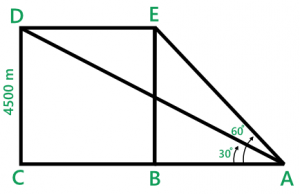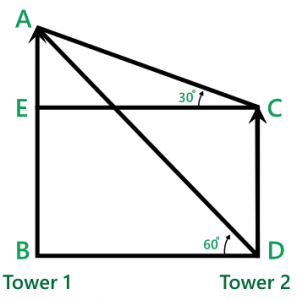Open in App
Not now

# Trigonometry & Height and Distances | Set-2

• Difficulty Level : Hard
• Last Updated : 16 Sep, 2021

Question 1: Find the maximum value of (2sinθ + 3 cosθ)
Solution : Max value of (asinθ + bcosθ) = √(a2 + b2))
So, max value = √(22 + 32
= √(4 + 9)
= √13

Question 2: Find the minimum value of the 4tan2θ + 9cot2θ
Solution : Minimum value = √ab
Here a = 4 and b = 9
Minimum value = √(4×9)
= √36
= 6

Question 3: If θ be an acute angle and 7sin2θ + 3cos2θ = 4, then the value of tanθ is
Solution : 7sin2θ + 3cos2θ = 4
7sin2θ + 3(1-sin2θ) = 4
7sin2θ + 3 – 3sin2θ = 4
4sin2θ = 1
sin2θ = 1/4
sinθ = 1/2
So, θ = 30o
then tanθ = 1/√3

Question 4:is equal to
Solution : It can be solved by rationalizationUse the property:-
(1-sin2θ = cos2θ)= 2/cosθ = 2 secθ

Question 5: If cosα = a cosβ and sinα = b sinβ, then the value of sin2β in terms of a and b is:
Solution : On squaring both sides
cos2α = a2 cos2β
=> 1 – sin2α = a2(1 – sin2β) ……..(1)
Again, sinα = b sinβ
On squaring both sides
sin2α = b2 sin2β
Put the value of sin2α in (1)
1 – b2 sin2β = a2 – a2sin2β)
a2 – 1 = a2sin2β – b2 sin2β
a2 – 1 = sin2β(a2 – b2
sin2β = a2 – 1 / (a2 – b2

Question 6: The value of tan1o tan2o tan3o…….tan89o is
Solution : Arrange such that A + B = 90o
(tan1otan89o)(tan2otan88o)…..(tan44otan46o)tan45o
= 1x1x1……1×1
= 1

Question 7: If sin 2A = cos(A – 15o), where 2A is an acute angle then the value of A is
Solution : sin 2A = cos(A – 15o
2A + A – 15o = 90o
3A = 105o
A = 35o

Question 8:then the value of sinθ is
Solution : By componendo and dividendo
tanθ/cotθ = 3/1
=> sin2θ = 3cos2θ
=> sin2θ = 3(1 – sin2θ)
=> 4sin2θ = 3
=> sin2θ = 3/4
sinθ = √/2

Question 9: If tan (π/2 – θ/2) = √3 then the value of cosθ is
Solution : tan (π/2 – θ/2) = √3
=> tan (90 – θ/2) = √3
=> cotθ/2 = √3 = cot 30o
=> θ/2 = 30o
=> θ = 60o
then cosθ = cos60o = 1/2

Question 10: a, b, c are the lengths of three sides of a triangle ABC. If a, b, c are related by the relation a2 + b2 + c2 = ab + bc + ca, then the value of (sin2A + sin2B + sin2C) is
Solution : Acc. to question
a2 + b2 + c2 – ab – bc – ca = 0
=>2a2 + 2b2 + 2c2 – 2ab – 2bc – 2ca = 0
=>(a-b)2 + (b-c)2 + (c-a)2 = 0
=> a=b=c
All three sides are equal then it is equilateral triangle.
then ∠A = ∠B = ∠C = 602
So, sin260 + sin260 + sin260
= 3(√3/2)2
= 9/4

Question 11: The angle of elevation of an aeroplane from a point on the ground is 30o. After flying for the 30 seconds, the elevation changes to 60o. If the aeroplane is flying at height of 4500 meters, then the speed of the aeroplane in km/hr is
Solution :In ∆ABE
tan 60 = P/B = 4500/AB
√3 = 4500/AB
AB = 4500/√3

In ∆ACD
tan 30 = 4500/AC
1/√3= 4500/AC
AC = 4500√3

Distance covered by plane in 30 seconds = AC – AB
=4500√3 – 4500/√3
= (13500 – 4500)/√3
= 9000/√3
= 3000√3

Speed= Distance/time = 3000√3 / 30
= 100√3 x 18/5 (to get km/hr)
= 360√3
Hence, speed of the plane is 360√3 km/hr

Question 12: There are two towers, one on each side of the road just opposite to each other. One tower is 54m high. From the top of this tower, the angles of the depression of the top and foot of the other tower are 30 and 60 respectively. The height of the other tower is:
Solution :AB and CD are Towers.
BD is the width of the road.
AB = 54 m
In ∆ AEC
tan 30 = AE/EC = 1/√3
=> AE : EC = 1 : √3
In ∆ABD
tan 60 = AB/BD
√3 = AB/BD
=> AB : BD = √3 : 1
From diagram we know EB = CD and EC = BD
Now,

AB     :     BD     :     AE
√3           1
√3     :      1
3      :     √3     :     1

CD = AB – AE = 3 – 1 = 2 units

3 units of AB -> 54 m
1 unit -> 18
Then 2 units -> 36 m
Hence, the height of the other tower is 36m.

My Personal Notes arrow_drop_up